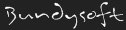L3DT documentationLarge 3D terrain generator

# Differences

This shows you the differences between two versions of the page.

Line 1: Line 1:
+====== Mask maps and generators ======
+
+
+//Mask maps// are greyscale images, such as that shown below, that are used in game engines to mask user-defined quantities such as grass coverage, vehicle accessibility, tiberium density, etc. A mask map is typically an 8-bit greyscale image.
+
+<html><div align = "center"></html>
+^  An example mask map, for grass coverage.  ^
+<html></div></html>
+
+
+In L3DT, //mask generators// are objects that create a mask maps. They do this by calculating a 'score' at each pixel using a set of parameters and weightings for terrain altitude and slope (gradient), water depth and salinity, and land type coverage. The parameters in a mask generator parameters may be viewed and edited using the [[l3dt:userguide:res:masks:maskedit|mask editor window]], shown below:
+
+<html><div align = "center"></html>
+^   The 'mask editor' window.  ^
+<html></div></html>
+
+==== Minimum, maximum and ramp settings ====
+
+For each of the //altitude//, //gradient//, //water depth// and //salinity// parameters, there is defined a minimum and maximum value, as well as a 'ramp' for each, and a weighting for the overall parameters.
+
+The meaning of the minimum, maximum and ramp values shall now be explained using the altitude settings in the above example image:
+  * The maximum value checkbox is enabled, and the maximum value was set to 1000 metres. This means the mask map will be 0 (black) for all terrain with an altitude of 1000m or higher.
+  * The maximum ramp has been set to 200 metres. This means that the mask map value will begin ramping down 200m below the maximum altitude. In this case, the score will be 100% at 800 metres (the beginning of the ramp), 50% at 900 metres (the mid-point of the ramp), and 0% at 1000 metres (the end of the ramp, at the maximum limit).
+  * The minimum value has been disabled, so this mask will not be affected by terrain altitudes lower than the max/ramp altitudes.
+
+==== Parameter weighting ====
+
+<note warning>
+This section is under construction! FIXME
+</note>
+
+==== Land type settings ====
+
+<note warning>
+This section is under construction! FIXME
+</note>
+
+==== Further calculation details ====
+
+For each mask parameter, a score between 0 and 1 is generated. The total score is calculated from the product of the scores of each mask parameter, and is then rescaled to a range of 0...255 for storage as an 8-bit image.